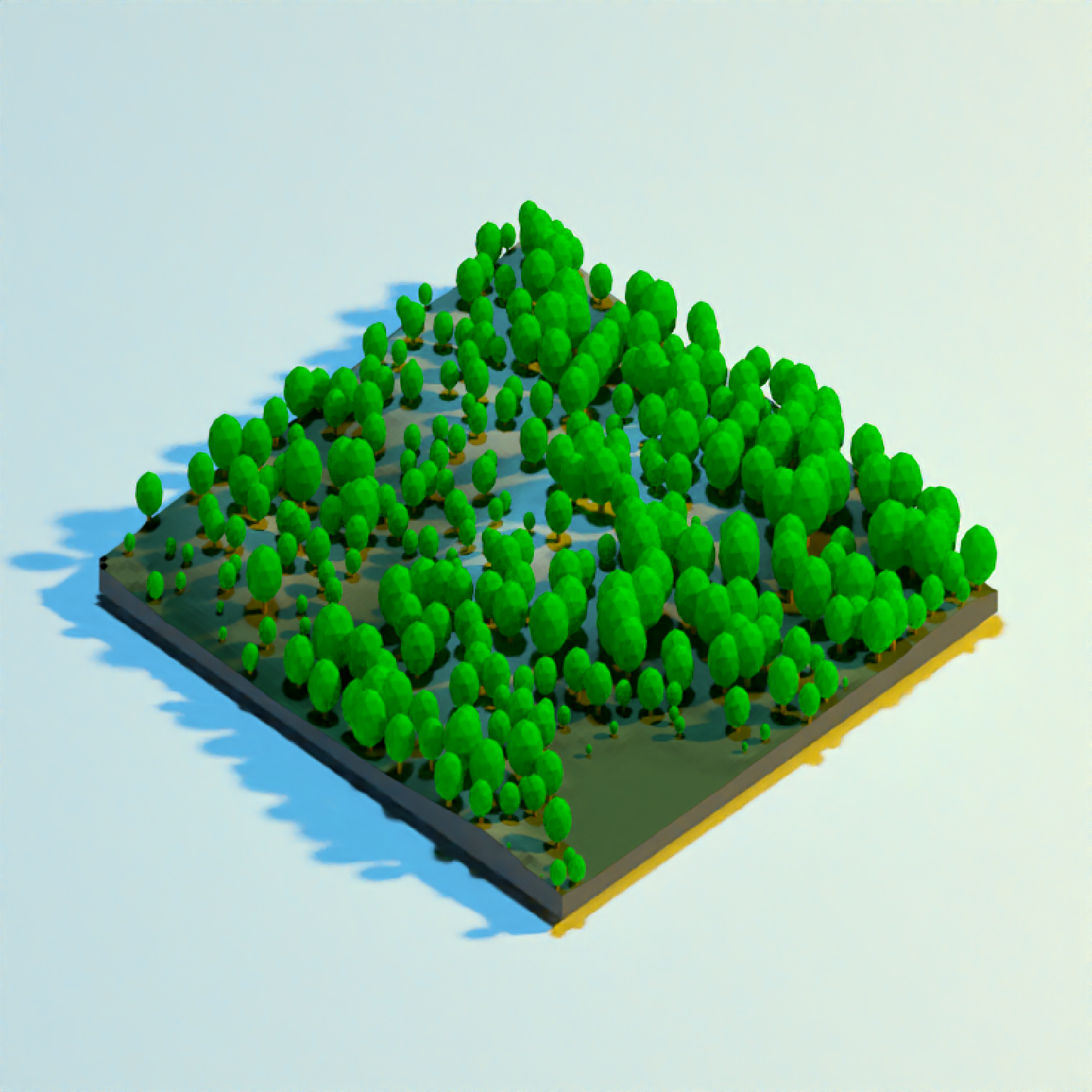Adds 3D tree to the current scene, using latitude/longitude or coordinates in the reference system defined by the extent object.

render_tree(
lat = NULL,
long = NULL,
extent = NULL,
type = "basic",
canopy_color = "#22aa22",
trunk_color = "#964B00",
absolute_height = FALSE,
canopy_height = 9,
canopy_width_ratio = NULL,
trunk_height = NULL,
tree_zscale = TRUE,
min_height = 0,
max_height = Inf,
zscale = 1,
heightmap = NULL,
baseshape = "rectangle",
angle = c(0, 0, 0),
clear_previous = FALSE,
...
)

## Arguments

lat

Vector of latitudes (or other coordinate in the same coordinate reference system as extent).

long

Vector of longitudes (or other coordinate in the same coordinate reference system as extent).

extent

Either an object representing the spatial extent of the 3D scene (either from the raster, terra, sf, or sp packages), a length-4 numeric vector specifying c("xmin", "xmax", "ymin", "ymax"), or the spatial object (from the previously aforementioned packages) which will be automatically converted to an extent object.

type

Default "basic". Type of tree. Other built-in option: "cone".

canopy_color

Default "darkgreen". Color(s) of the canopy.

trunk_color

Default "#964B00" (brown). Color(s) of the trunk,

absolute_height

Default FALSE. Default is specifying the tree height directly, relative to the underlying height map. If TRUE, canopy_height will specified by the actual altitude of the top of the tree. Total tree height will be canopy_height + trunk_height.

canopy_height

Default 9. Height of the canopy, in units of height map. Total tree height will be canopy_height + trunk_height.

canopy_width_ratio

Default 1. Ratio of the canopy width to the canopy height. 1 is spherical.

trunk_height

Default NULL, automatically computed. Height of the trunk, from the ground. Default is 1/3rd the canopy height if type = "basic", and 1/6th the canopy height if type = "cone". Total tree height will be canopy_height + trunk_height.

Default NULL, automatically computed. Default is 1/5rd the trunk height if type = "basic", and 1/10th the trunk height if type = "cone".

tree_zscale

Default TRUE. Whether to scale the size of the tree by zscale to have it match the size of the map. If zscale is very big, this will make the trees very small.

min_height

Default NULL. Minimum height of a tree. Set to a positive number to filter out trees below that height.

max_height

Default NA. Maximum height of a tree. Set to a positive number to filter out trees above that height.

zscale

Default 1. The ratio between the x and y spacing (which are assumed to be equal) and the z axis in the original heightmap.

heightmap

Default NULL. Automatically extracted from the rgl window--only use if auto-extraction of matrix extent isn't working. A two-dimensional matrix, where each entry in the matrix is the elevation at that point. All points are assumed to be evenly spaced.

baseshape

Default rectangle. Shape of the base. Options are c("rectangle","circle","hex").

angle

Default c(0,0,0). Angle of rotation around the x, y, and z axes. If this is a matrix or list, each row (or list entry) specifies the rotation of the nth tree specified (number of rows/length of list must equal the length of lat/long).

clear_previous

Default FALSE. If TRUE, it will clear all existing trees.

...

Additional arguments to pass to rgl::triangles3d().

## Examples

if(rayshader:::run_documentation()) {
#Let's first start by drawing some trees in a circle around Monterey Bay
#We won't scale these to a realistic size (yet)
moss_landing_coord = c(36.806807, -121.793332)
montereybay %>%
plot_3d(montereybay,zscale=50,water=TRUE,
theta=210,  phi=22, zoom=0.20, fov=55)

t = seq(0,2*pi,length.out=20)
circle_coords_lat = moss_landing_coord + 0.3 * sin(t)
circle_coords_long = moss_landing_coord + 0.3 * cos(t)

render_tree(extent = attr(montereybay,"extent"), heightmap = montereybay,
tree_zscale = FALSE, canopy_height = 30, canopy_width_ratio = 1,
lat = unlist(circle_coords_lat), long = unlist(circle_coords_long), zscale=50)
render_snapshot()
}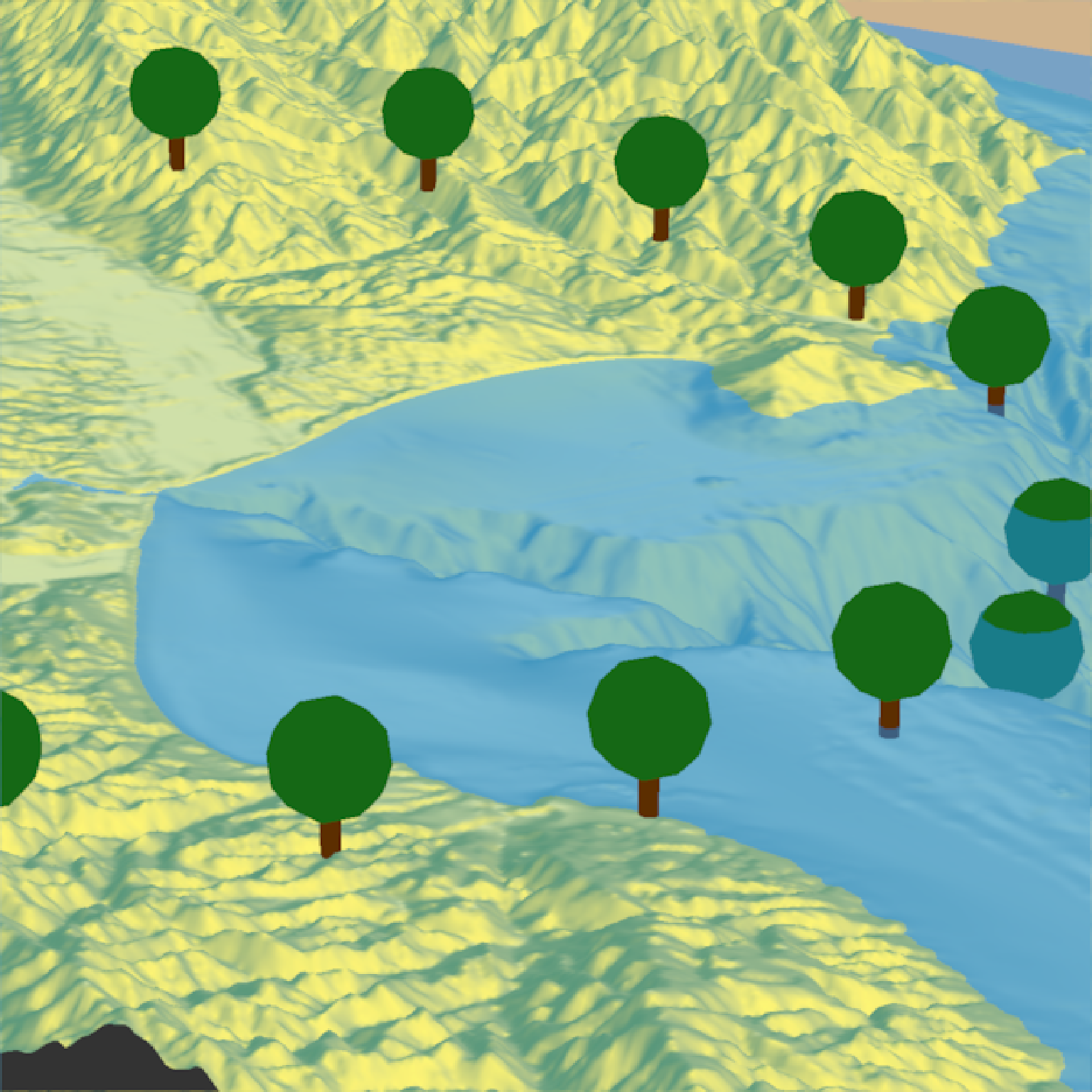#Change the canopy width ratio (compared to the height)
render_tree(extent = attr(montereybay,"extent"), heightmap = montereybay,
tree_zscale = FALSE, canopy_height = 30, canopy_width_ratio = 0.5,
clear_previous = TRUE,
lat = unlist(circle_coords_lat), long = unlist(circle_coords_long), zscale=50)
render_snapshot()
}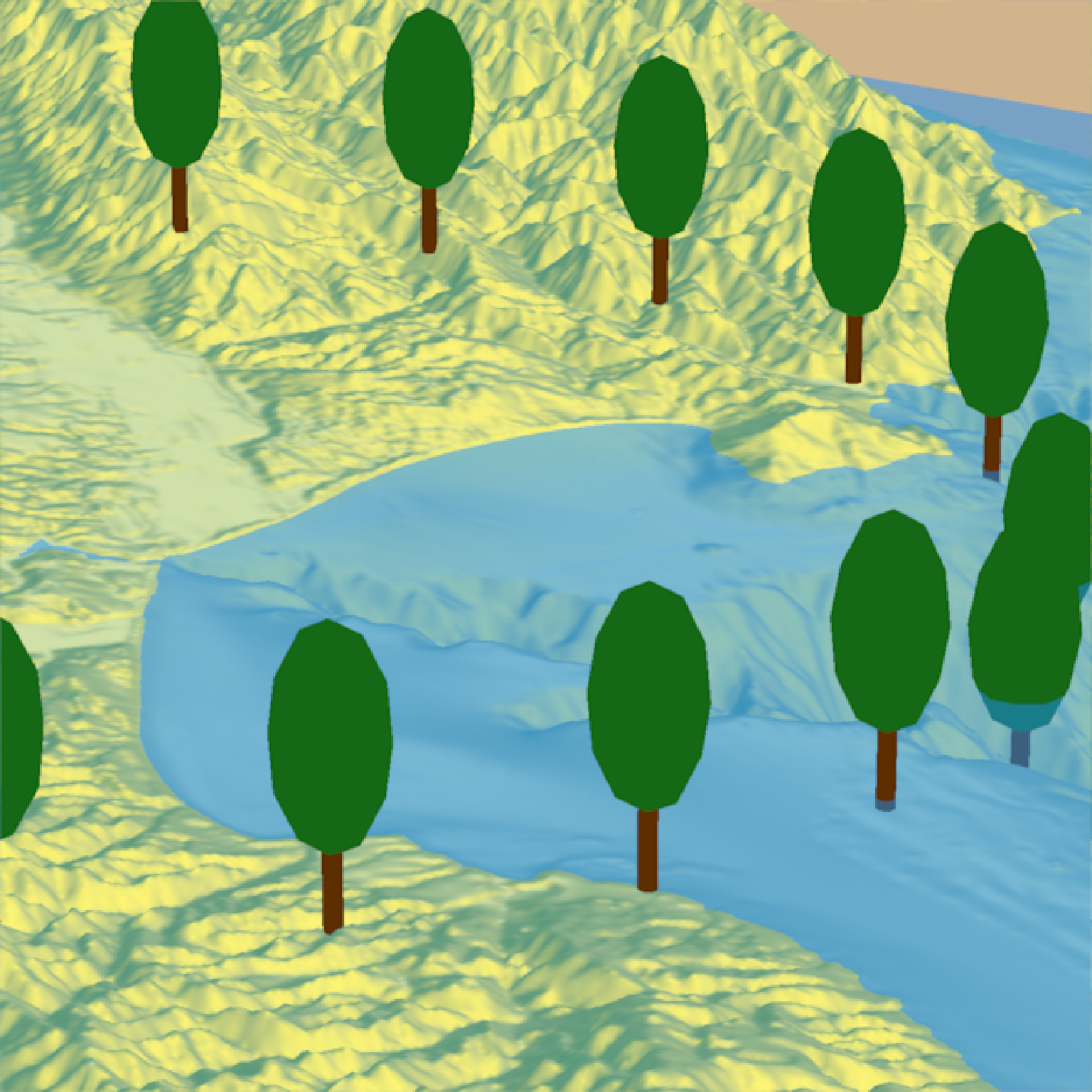#Change the trunk height and width
render_tree(extent = attr(montereybay,"extent"), heightmap = montereybay,
tree_zscale = FALSE, canopy_height = 10, canopy_width_ratio = 2,
clear_previous = TRUE, trunk_height=15, trunk_radius = 1.5,
lat = unlist(circle_coords_lat), long = unlist(circle_coords_long), zscale=50)
render_snapshot()
}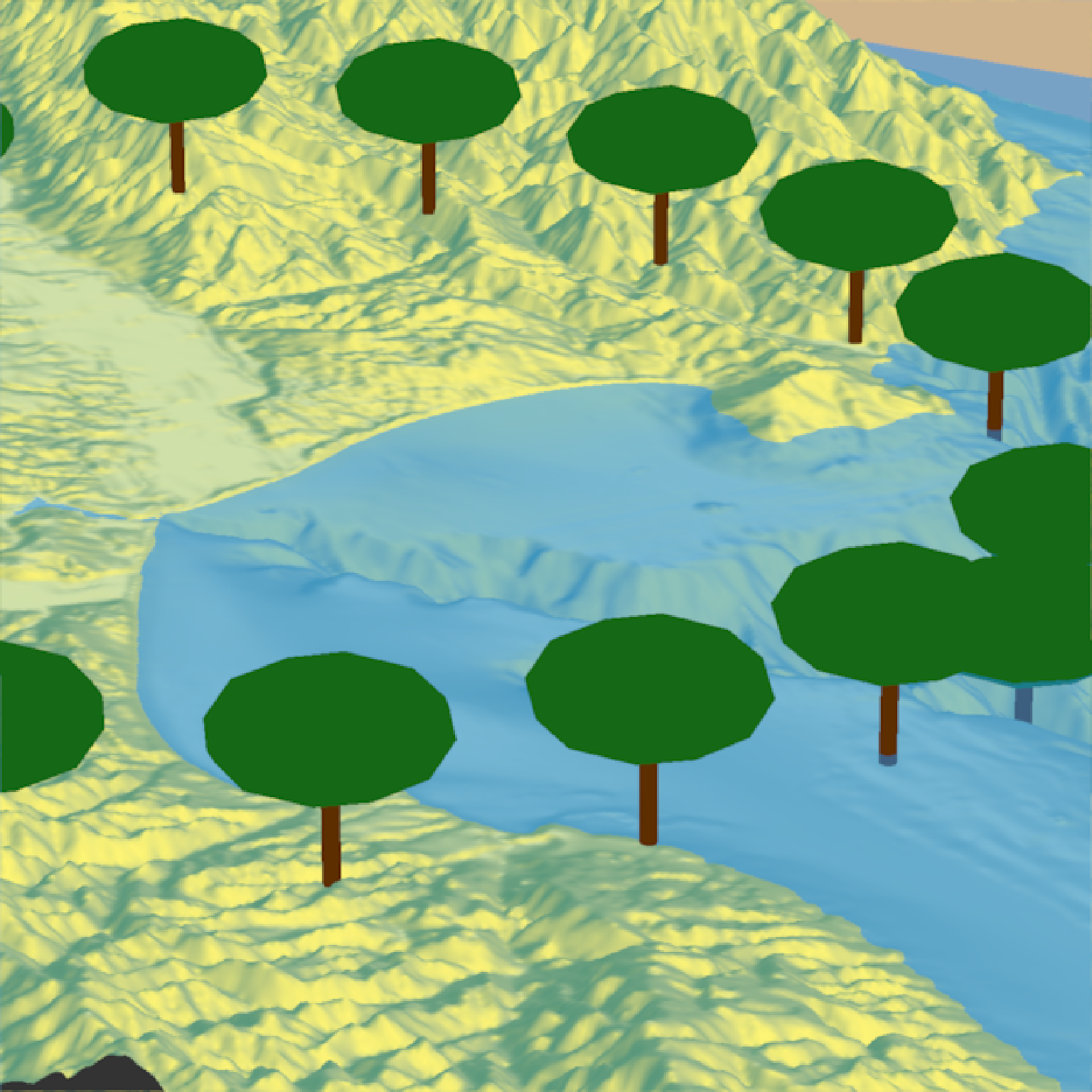#Change the tree type
render_tree(extent = attr(montereybay,"extent"), heightmap = montereybay,
tree_zscale = FALSE, canopy_height = 30,
clear_previous = TRUE, type = "cone",
lat = unlist(circle_coords_lat), long = unlist(circle_coords_long), zscale=50)
render_snapshot()
}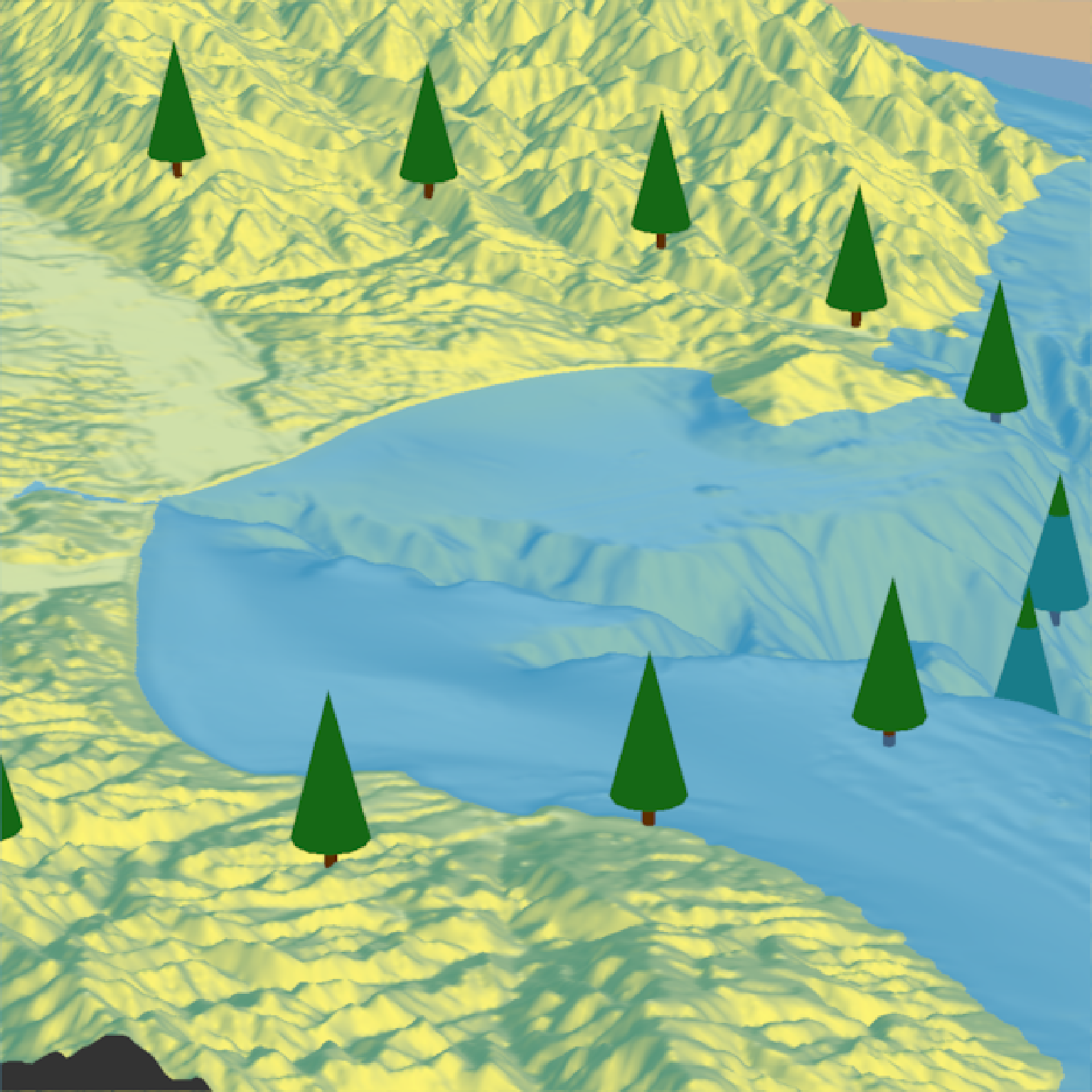#Change the canopy color:
render_camera(theta = 150,  phi = 38, zoom = 0.4, fov = 55)
render_tree(extent = attr(montereybay,"extent"), heightmap = montereybay,
tree_zscale = FALSE, canopy_height = 30, canopy_width_ratio = 1,
canopy_color = rainbow(20),  trunk_height=20,
clear_previous = TRUE,
lat = unlist(circle_coords_lat), long = unlist(circle_coords_long), zscale=50)
render_snapshot()
}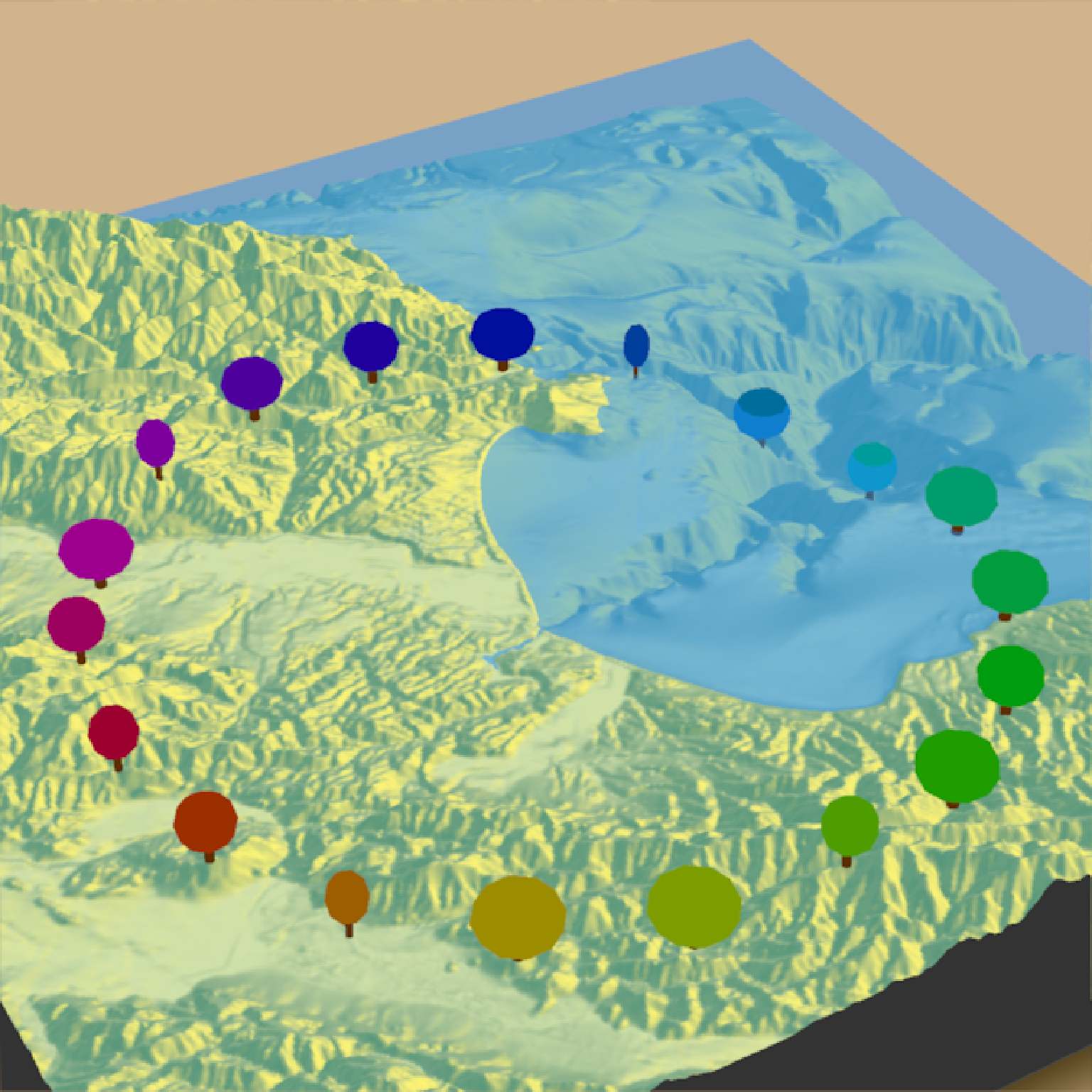#We will use the lidR package to generate a DEM and detect the canopy tops of trees, and
#then use rayshader to render 3D tree models scaled to those heights on the map.
run_example = length(find.package("lidR", quiet = TRUE)) > 0 &&
length(find.package("sf", quiet = TRUE)) > 0 &&
length(find.package("terra", quiet = TRUE)) > 0 &&
if (run_example) {
#Load the example data from the lidR package
LASfile = system.file("extdata", "Topography.laz", package="lidR")
las = lidR::readLAS(LASfile, filter = "-inside 273450 5274350 273550 5274450")

#Convert the lidar point data to a DEM and detect the location of trees from the same data
dem = lidR::rasterize_terrain(las, algorithm = lidR::tin())
tree_top_data = lidR::locate_trees(las, lidR::lmf(ws = 5))
tree_locations = sf::st_coordinates(tree_top_data)

#Convert DEM to a matrix and extract the extent of the scene
dem_matrix = raster_to_matrix(dem)
dem_extent = terra::ext(dem)
extent_values = dem_extent@ptr\$vector

#Plot the ground
dem_matrix |>
plot_3d(dem_matrix)
render_snapshot()
}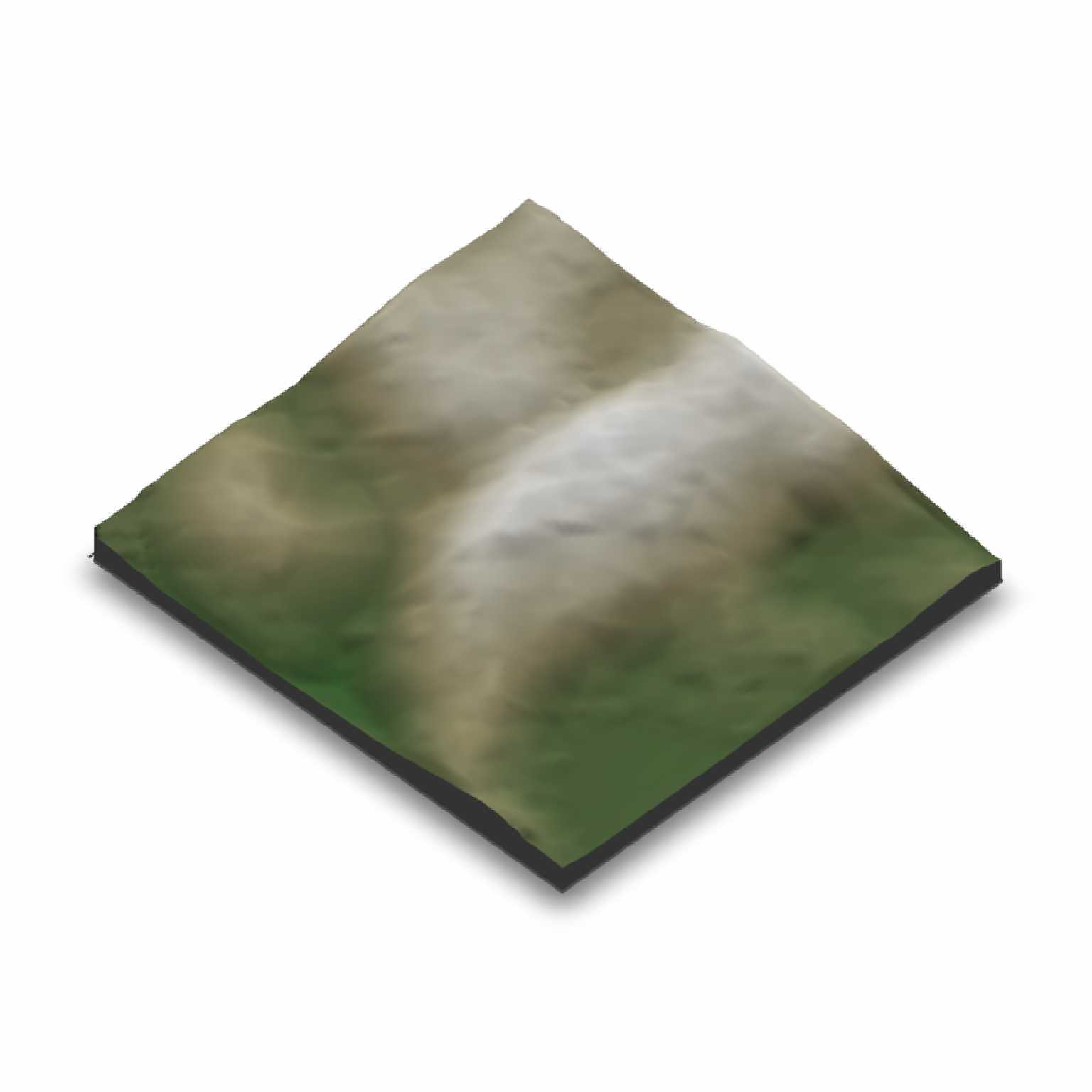if (run_example) {
#The tree locations are given as an absolute height (as opposed to relative to the surface)
#so we set absolute_height = TRUE.
render_tree(lat = tree_locations[,2], long = tree_locations[,1],
canopy_width_ratio = 0.5, clear_previous = T,
absolute_height = TRUE, canopy_height = tree_locations[,3],
canopy_color = "#00aa00",
extent = raster::extent(extent_values), heightmap = dem_matrix)

#Remove existing lights and add our own with rgl
rgl::pop3d("lights")
rgl::light3d(phi=35,theta=90, viewpoint.rel=F, diffuse="#ffffff", specular="#000000")
rgl::light3d(phi=-45,theta=-40, viewpoint.rel=F, diffuse="#aaaaaa", specular="#000000")
render_snapshot()
}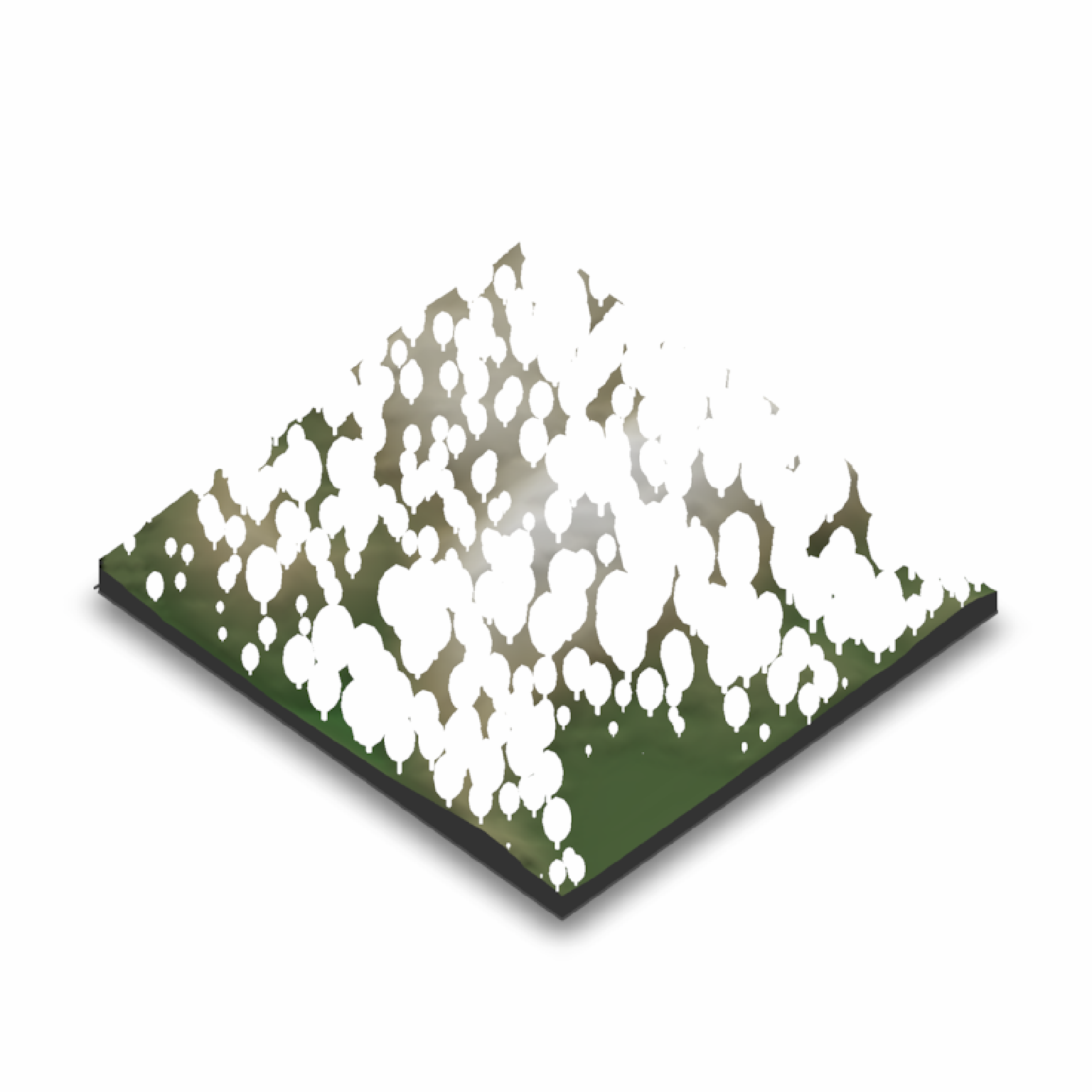if (run_example) {
#Render tree also works with render_highquality()
render_highquality(lightdirection=c(90,45),lightaltitude=c(90,45),
lightcolor=c("dodgerblue","orange"),
min_variance = 0, sample_method="sobol_blue", clamp_value=10)
}